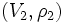# Finite-dimensional linear representation

Jump to: navigation, search

This article describes a property to be evaluated for a linear representation of a group, i.e. a homomorphism from the group to the general linear group of a vector space over a field

This article gives a basic definition in the following area: linear representation theory
View other basic definitions in linear representation theory |View terms related to linear representation theory |View facts related to linear representation theory

## Definition

### Symbol-free definition

A linear representation of a group is termed finite-dimensional if its vector space is finite-dimensional as a vector space over the field.

### Definition with symbols

Let$G$ be a group and$k$ a field. A finite-dimensional linear representation of$G$ over$k$ is a homomorphism$\rho:G \to GL(V)$ where$V$ is a finite-dimensional vector space over$k$. The representation is typically expressed by the pair$(V,\rho)$.

### Equivalence of representations

Further information: equivalent linear representations

The notion of equivalence of finite-dimensional linear representations is the same as the notion of equivalence as linear representations. Namely, given two finite-dimensional linear representations$(V_1,\rho_1)$ and$(V_2,\rho_2)$ of$G$, an equivalence between them is a map$\sigma: V_1 \to V_2$ such that for all$g$:$\rho_2(g) = \sigma \circ \rho_1(g) \circ \sigma^{-1}$

In particular, when$V_1 = V_2 = V$, then two representations on$V$ are equivalent if they are conjugate by an element of$GL(V)$.

## Invariants

If$(V,\rho)$ is a finite-dimensional linear representation of$k$, and$V$ has dimension$n$, there is an isomorphism$\sigma$ between$V$ and$k^n$ (i.e., a choice of basis for$V$). Define a representation$\rho'$ on$k^n$ as$\sigma \circ \rho \circ \sigma^{-1}$. Then,$\rho'$ associates, to every element of$G$, an invertible$n \times n$ matrix.

### Conjugacy class of image

For$(V,\rho)$ a finite-dimensional linear representation of$G$, we can associate to every$g$, the conjugacy class of$\rho(g)$ in$GL(V)$. This conjugacy class is invariant under equivalence of representations, because any equivalence of representations involves conjugating by an element of$GL(V)$.

### Trace and character

Further information: character of a representation

For$(V,\rho)$ a finite-dimensional linear representation of$G$, we can associate to every$g \in G$, the trace of$\rho(g)$. (In the concrete situation where$V = k^n$ and$\rho(g)$ is viewed as a matrix, this is the sum of the diagonal entries of$\rho(g)$). Since the trace depends only on the conjugacy class of the representation, it is invariant under equivalence of representations.

The function sending each element of the group to its trace is termed the character of the representation. The character is thus a function from the group to the field.

### Degree

Further information: Degree of a representation

The degree of a finite-dimensional linear representation, also called the dimension of the representation, is the dimension of the vector space on which it acts. Equivalently, it is the character evaluated at the identity element of the group.

## Constructions

### Direct sum

Further information: Direct sum of linear representations

Let$(V_1,\rho_1)$ and$(V_2,\rho_2)$ be two finite-dimensional linear representations. Then, the direct sum of these is given as:

• The vector space for it is$V_1 \oplus V_2$
• The action is:$g.(v_1,v_2) = (g.v_1, g.v_2)$

In other words$G$ acts component-wise.

If we are thinking of both representations in terms of matrices, i.e.$V_1 = k^n$ and$V_2 = k^m$, then$(\rho_1 \oplus rho_2)(g)$ is a block matrix looking like:$\begin{pmatrix} \rho_1(g) & 0 \\ 0 & \rho_2(g) \end{pmatrix}$

### Tensor product

Further information: Tensor product of linear representations

PLACEHOLDER FOR INFORMATION TO BE FILLED IN: [SHOW MORE]